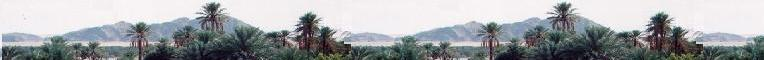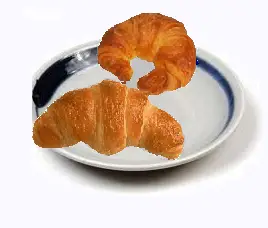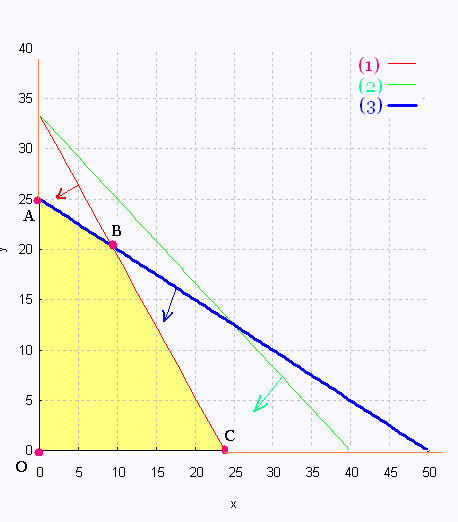# Mathematics 2: Linear optimization Examples

### CrescentsA baker makes two types of crescents:
He has 20 kg of butter, 4 kg sugar, and 2 kg of egg yolk (100 eggs).

Type A : extra butter:
840 g butter,
100 g of sugar,
40 g egg yolk.

This recipe produces 10 crescents, $15 each. Type B extra eggs: 600 g butter, 120 g of sugar, 80 g egg yolk This recipe produces 10 crescents,$ 18 each.

How many of each kind of recipes the baker must make to have a maximum profit. What is this profit?

x number of recipes A
y number of recipes B

Butter: 840 x + 600 y = 20,000
Sugar: 100 x + 120 y = 4000
Yolk: 40 x + 80 y = 2000

(1): 21x + 15y = 500
(2): 5x + 6y = 200
(3): x + 2y = 50

(1): y = - 7x/ 5 + 100/3
(2): y = - 5x/ 6 + 100/3
(3): y = - x/ 2 + 25

Z = 15 x + 18 y maximumThe 4 vertices are:

1. O(0,0)

2. A(0, 25)

3. C(500/21,0)


4. Let's find the coordinates of the point B of the intersection of the two lines (1) and (3):

Equating the two related equations gives:

- 7x / 5 + 100/3 = - x/2 + 25

Solving for x leads to the following repeating decimals:

x = 250/27 = 9.259 and y = 550/27 = 20.370.

The value of the function objective Z is then:

Z = 15 (250/27) + 18 (550/27 ) = 4550/9 = 505.5

Note that x number of recipes A, and y number of recipes B. These numbers connot be, in this contexte, decimal numbers. We need to round them.

We cannot round up the values of x and y, because we will need more the given ingredients.

Rounding down, we get:

x = 9 and y = 20.

The value of the function objective Z is then:

Z = 15 (9) + 18 (20) = 495.00 $. In this case the related loss is: 4550/9 - 495 = 95/9 = 10.5$

We have to accept this loss we don't have a better choice.

 Vertex Z ($) (0, 0) 0 (500/21, 0) 357.14 (9, 20) 495$ ***     →     loss: 10.5 $(0, 25) 450 To obtain the maximum profit, which is 495$, along with a loss of about 11 \$, the baker will make 9 recipes of crescent type A and 20 recipes of crescent type B.Web ScientificSentence

 chimie labs | scientific sentence | java | php | green cat | contact |